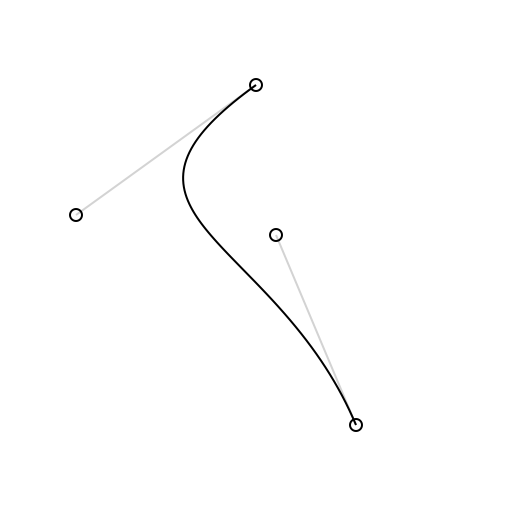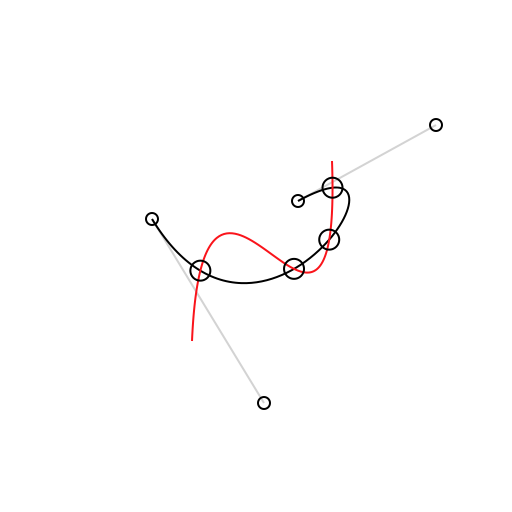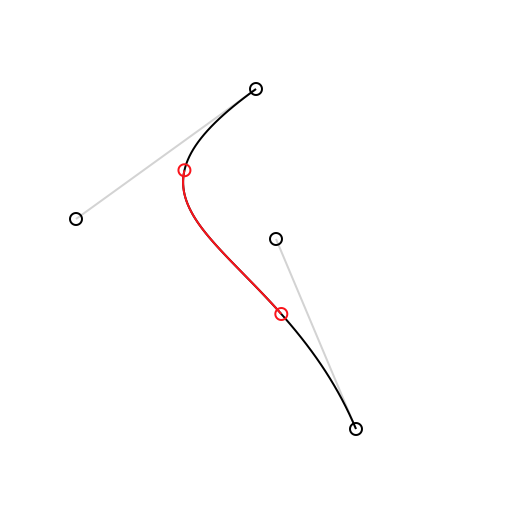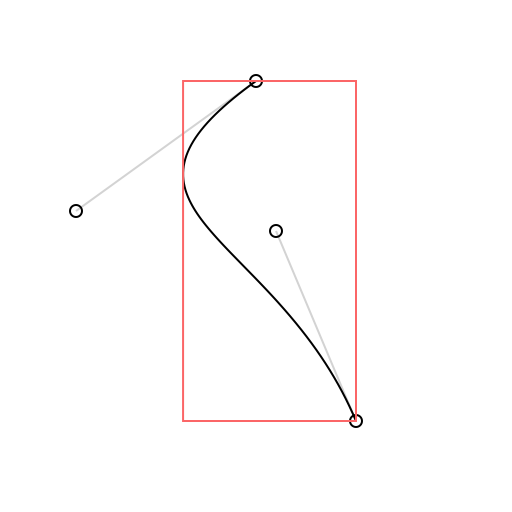Latest 0.5.8 https://github.com/hfutrell/BezierKit MIT ios 10.0, osx 10.12 Holmes Futrell

BezierKit is a comprehensive Bezier Path library written in Swift.

## Warning! Prerelease software!

Please note that BezierKit is currently pre-release software. Its releases follow semantic versioning which means that until it reaches 1.0 status the API may not be stable or backwards compatible.

## Features

• [x] Constructs linear (line segment), quadratic, and cubic Bézier curves
• [x] Draws curves via CoreGraphics
• [x] Determines positions, derivatives, and normals along curves
• [x] Lengths of curves via Legendre-Gauss quadrature
• [x] Intersects curves and computes cubic curve self-intersection to any degree of accuracy
• [x] Determines bounding boxes, extrema,
• [x] Locates nearest on-curve location to point
• [x] to any degree of accuracy
• [x] Splits curves into subcurves
• [x] Offsets and outlines curves
• [ ] Comprehensive Unit and Integration Test Coverage
• [ ] Complete Documentation

## Installation with CocoaPods

The recommended way to install BezierKit is via CocoaPods, however you may also find that dropping the contents of `Library` into your project also works.

CocoaPods is a dependency manager for Cocoa projects. You can install it with the following command:

``\$ gem install cocoapods``

To integrate BezierKit into your Xcode project using CocoaPods, add it to your target in your `Podfile`:

``````target '<Your Target Name>' do
pod 'BezierKit', '>= 0.5.10'
end``````

Then, run the following command:

``\$ pod install``

## Usage

### Constructing & Drawing Curves

BezierKit supports cubic Bezier curves (`CubicBezierCurve`) and quadratic Bezier curves (`QuadraticBezierCurve`) as well as line segments (`LineSegment`) each of which adopts the `BezierCurve` protocol that encompasses most API functionality.``````import BezierKit

let curve = CubicBezierCurve(
p0: CGPoint(x: 100, y: 25),
p1: CGPoint(x: 10, y: 90),
p2: CGPoint(x: 110, y: 100),
p3: CGPoint(x: 150, y: 195)
)

let context: CGContext = ...       // your graphics context here
Draw.drawSkeleton(context, curve)  // draws visual representation of curve control points
Draw.drawCurve(context, curve)     // draws the curve itself``````

### Intersecting Curves

The `intersections(with curve: BezierCurve) -> [Intersection]` method determines each intersection between `self` and `curve` as an array of `Intersection` objects. Each intersection has two fields: `t1` represents the t-value for `self` at the intersection while `t2` represents the t-value for `curve` at the intersection. You can use the `compute(_:)` method on either of the curves to calculate the coordinates of the intersection by passing in the corresponding t-value for the curve.

Cubic curves may self-intersect which can be determined by calling the `selfIntersections()` method.``````let intersections: [Intersection] = curve1.intersections(with: curve2)
let points: [CGPoint] = intersections.map { curve1.compute(\$0.t1) }

Draw.drawCurve(context, curve: curve1)
Draw.drawCurve(context, curve: curve2)
for p in points {
Draw.drawPoint(context, origin: p)
}``````

### Splitting Curves

The `split(from:, to:)` method produces a subcurve over a given range of t-values. The `split(at:)` method can be used to produce a left subcurve and right subcurve created by splitting across a single t-value.``````Draw.setColor(context, color: Draw.lightGrey)
Draw.drawSkeleton(context, curve: curve)
Draw.drawCurve(context, curve: curve)
let subcurve = curve.split(from: 0.25, to: 0.75) // or try (leftCurve, rightCurve) = curve.split(at:)
Draw.setColor(context, color: Draw.red)
Draw.drawCurve(context, curve: subcurve)

### Determining Bounding Boxes``````let boundingBox = curve.boundingBox
Draw.drawSkeleton(context, curve: curve)
Draw.drawCurve(context, curve: curve)
Draw.setColor(context, color: Draw.pinkish)
Draw.drawBoundingBox(context, boundingBox: curve.boundingBox)``````

### More

BezierKit is a powerful library with a lot of functionality. For the time being the best way to see what it offers is to build the MacDemos target and check out each of the provided demos.

### Latest podspec

```{
"name": "BezierKit",
"version": "0.5.8",
"summary": "comprehensive Bezier Path library written in Swift",
"homepage": "https://github.com/hfutrell/BezierKit",
"authors": {
"Holmes Futrell": "[email protected]"
},
"platforms": {
"ios": "10.0",
"osx": "10.12"
},
"ios": {
"frameworks": [
"UIKit",
"CoreGraphics"
]
},
"osx": {
"frameworks": "AppKit"
},
"source": {
"git": "https://github.com/hfutrell/BezierKit.git",
"tag": "v0.5.8"
},
"source_files": "BezierKit/Library"
}```